Courses

# Test: Stereochemistry- 1

## 20 Questions MCQ Test Organic Chemistry | Test: Stereochemistry- 1

Description
This mock test of Test: Stereochemistry- 1 for Chemistry helps you for every Chemistry entrance exam. This contains 20 Multiple Choice Questions for Chemistry Test: Stereochemistry- 1 (mcq) to study with solutions a complete question bank. The solved questions answers in this Test: Stereochemistry- 1 quiz give you a good mix of easy questions and tough questions. Chemistry students definitely take this Test: Stereochemistry- 1 exercise for a better result in the exam. You can find other Test: Stereochemistry- 1 extra questions, long questions & short questions for Chemistry on EduRev as well by searching above.
QUESTION: 1

Solution:
QUESTION: 2

Solution:
QUESTION: 3

### Which type of conformation is shown by I and II: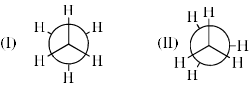Solution:
QUESTION: 4

The most stable conformation of ethylene glycol is:

Solution:
QUESTION: 5

In the boat conformation of cyclohexane, the most destabilizing interaction is:

Solution:

Eclipsed atoms are not valid in boat configurations.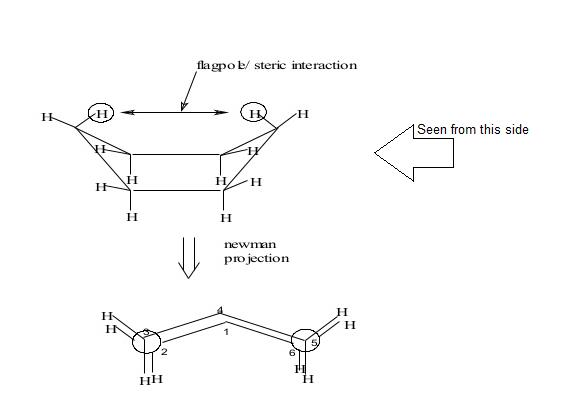Flagpole interaction generally raises the strain and then energy in cyclohexane (boat conformational structure). You can imagine this as two hydrogen atoms at pole position (1, 4) and the interaction between them.

The boat conformations have higher energy than the chair conformations. The interaction between the two flagpole hydrogen atoms, in particular, generates steric strain. Torsional strain also exists between the C2–C3 and C5–C6 bonds, which are eclipsed. Because of this strain, the boat configuration is unstable (i.e. is not a local energy minimum).

Hence D is correct.

QUESTION: 6

The reaction in which stereochemically different molecules react differently is called

Solution:

The reaction in which stereochemically different molecules react differently is called stereospecific reaction. Stereospecificity is the property of a reaction mechanism that lead to different stereo isomeric reaction products from different stereo isomeric reactants, or which operates on only one of the stereo isomers.

QUESTION: 7

The configurations at the two stereocentres in the compound given below are: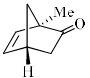Solution:
QUESTION: 8

The configuration at the two stereocentres in the compound given below are: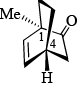Solution:
QUESTION: 9

The two compounds given below are: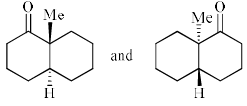Solution:
QUESTION: 10

The absolute configuration at the two chiral centers of (–)–camphor is: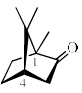Solution: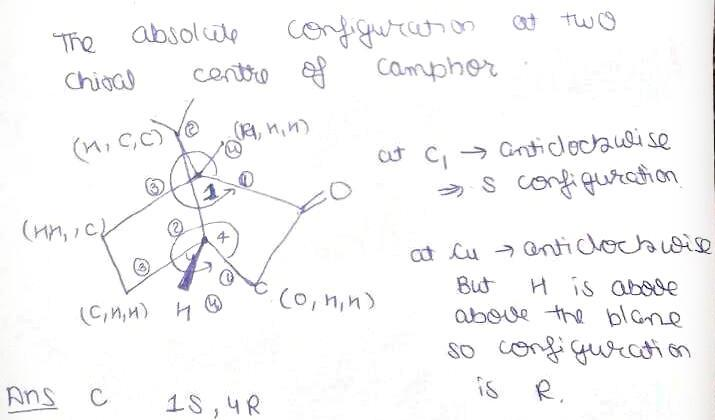Hence D is the correct answer.

QUESTION: 11

In the following Markonikov addition reaction, the products A and B are: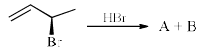Solution:
QUESTION: 12

Epoxidation of (R)-cyclohexene-2-ol with peracetic acid yields a 95:5 mixture of compounds A and B compounds A and B are:

Solution:
QUESTION: 13

Among the following the most stable isomer for 3-methoxycylohexanol is:

Solution:
QUESTION: 14

What is the relation between shown compounds: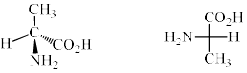Solution:
QUESTION: 15

Which of the following term best describes the pair of compound shown: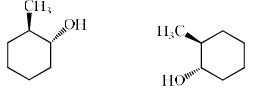Solution:
QUESTION: 16

The first person to separate a racemic mixture into individual enantiomers is:

Solution:
QUESTION: 17

Among the following dibromocyclohexanes, the one that reacts fastest with sodium iodide to give cyclohexene is:

Solution:
QUESTION: 18

Which of the following term best describes the pair of compound shown: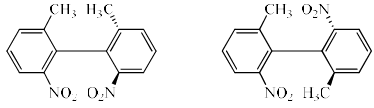Solution:
QUESTION: 19

Which structure is different from the following?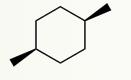Solution:

The two substituents in the cis 1,4-disubstituted chair cyclohexane shown are necessarily axial and equatorial, as in (a), (b) and (d). In (c), however, the 1,4-substituents are both equatorial so this is the trans isomer, i.e. a different compound.

QUESTION: 20

The correct order of stability for the following conformations of cyclohexane is: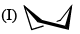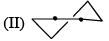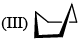Solution: